If you have a related question, please click the "Ask a related question" button in the top right corner. The newly created question will be automatically linked to this question.

# UCD90320: UCD90320 fusion design tool question

Part Number: UCD90320
Other Parts Discussed in Thread: INA210, TMP236,

Hi there

In fusion design tool, can you tell me what does each scaling function do? For example Vout Scal Mon, Vout Cal mon, Iout Cal mon, Iout Cal gain

And how do I know what value to enter for the first time?

We are using TI current sensor INA210 amplifier that connects to Amon pin for monitoring the power supply current.

I have posted another related question for INA210 as following link:

e2e.ti.com/.../ina210-ina210-question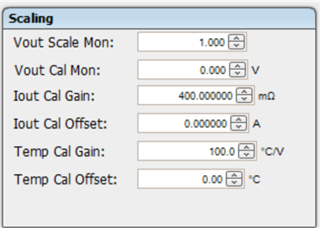Thanks

Mike Hsu

• HI

Vout Scale MON is the external voltage divider. the monitoring voltage of UCD is up to the ADC reference, any voltage larger than ADC reference need a external voltage divider. Here is the place to put ratio

VOUT CAL Mon: is the offset you want to add. for example, GND is not a 0V, it is 100mV or whatever, you can put here. typical it is 0.

Iout Cal Gain/Offset: you have to set this base on the INA and sensing resistor. Please be noted that UCD can only monitor voltage, device will convert the voltage back to current based on the gain and offset.

The same applies on the Temp cal gain and offset, the temp sensor's data sheet shall give your a formula to convert the voltage to temp based on the gain and offset

Regards

Yihe

• Hi

1. I have a voltage divider circuit Vin = 5V, Rup=4.99K ohm, Rdown=1k ohm on a Amon pin. The ratio is 0.2 right?   (1K ohm / 4.99k ohm = 0.2)

Vout Scale Mon = 0.2

2. There is no need for voltage divider circuit. For example 1.8V through current sensing amplifier (INA210). Is the ratio for Vout Scale Mon = 1.0

3. I am using TMP236 for anlog output temperature sensor.  Can I just enter 19.5mV/C for Temp cal gain and -10 celsius for Temp Cal Offset, see table 2?

4. How does UCD90320 know -10 celsius = 205mV ?

if the ambient temperature range is -10 celsius to +80 celsius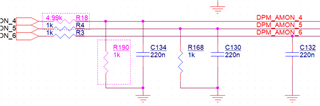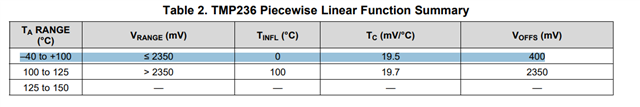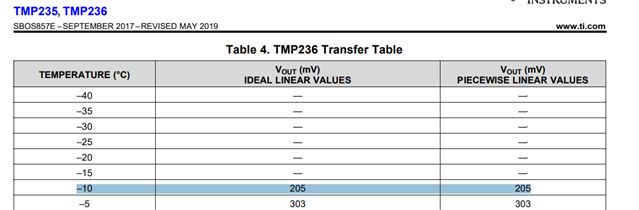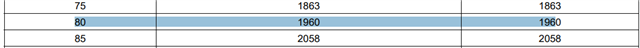• Hi

1. the ratio is bottom/(top + bottom)

2 for 1.8V, no divider is needed

3./4: UCD only monitor the voltage and convert the voltage to temp: temp = Vol * gain + offset.

Regards

Yihe

• For question 2 above, what is the Vout Scale Mon if there is no voltage divider?

Thanks

Mike

• Hi

If there is no divider, the ratio shall be set to 1.

Regards

Yihe